telescopeѲptics.net          ▪▪▪▪                                             CONTENTS

# 8.2.2. CLASSICAL TWO-MIRROR TELESCOPES

While spherical aberration, according to Eq. 81, can be corrected for any appropriate combination of the primary and secondary mirror conic, the aberration coefficients for coma and astigmatism for two-mirror system show that coma and astigmatism do vary, potentially significantly, with the choice of conics. The coma aberration coefficient (Eq. 82) indicates that a system with spherical primary will have stronger coma than one with paraboloidal primary. A particular value of K1 resulting in the zero sum in the brackets, would result in corrected Seidel coma, with needed value for the secondary conic for corrected spherical aberration obtained from Eq. 80.

In the early days of telescopes, limitations in both, calculation and mirror-making and testing skills would not allow to determine precisely the coma-free conic combination. However, it was determined that paraboloidal primary significantly reduces coma, compared to spherical (Eq. 82.1 shows that astigmatism with paraboloidal primary actually increases, but insignificantly in comparison with the reduction in coma). Thus, the choice for primary conic was, for quite some time, paraboloid and, for that reason, this arrangement is known as the classical two-mirror telescope.

With paraboloidal primary (K1=-1), which is corrected for spherical aberration, zero system spherical aberration requires zero secondary spherical aberration, for which the needed secondary mirror conic is, from Eq. 80: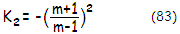which makes it a hyperboloid. Since the requirement in the classical two-mirror system is that either mirror has zero spherical aberration, mirror conic can be alternatively determined from the fact that the object for each has to be located at one of its geometric foci. For the paraboloidal primary, the object is at its infinity focus. For the secondary, the object is the image formed by the primary, thus it has to coincide with secondary mirror's near geometric focus (at the far focus location, the final image wouldn't be real, that is, the rays would be diverging from the secondary). Equaling the near focus separation for the secondary given by R2/(1+ε2), ε2 being the surface eccentricity, with the secondary-to-primary's-image separation i (FIG. 121), requires secondary mirror eccentricity ε2=(R2/i)-1, or the secondary conic constant K2=-[(R2/i)-1]2 where, according to the sign convention, for the primary oriented to the left, both secondary mirror radius of curvature R2 and image separation i are negative in the Cassegrain and positive in the Gregorian.

With the object at its near focus, secondary mirror will form the final image at its far focus, at a distance equal to R2/(1-ε2).

After spherical aberration is corrected, remaining Seidel coma and astigmatism, as the P-V wavefront error at diffraction focus for object at infinity are given by: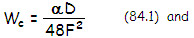respectively, with α being the field angle in radians, D the aperture diameter, F the system F-number and ƒ the system focal length. The coma RMS wavefront error is related to the P-V error as:

ω = Wc/32 = αD/272F2 = h/272F3          (84.3)

and for astigmatism:

ωa=Wa/241/2          (84.4)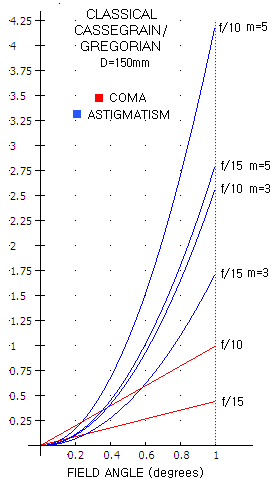Plots at left show the P-V wavefront error (WFE, in units of 550nm wavelength) for the two aberrations, based on Eq. 84.1 and 84.2. Coma only depends on the system F-ratio and aperture diameter, while the astigmatism also depends on secondary magnification and weakly on the back focal length (BFL, assumed to be 0.25, or one quarter of the primary's focal length). Astigmatism is nearly proportional to secondary magnification, while inversely proportional to the system F-ratio. In other words, for given focal ratio, it increases with faster primary. The combined RMS wavefront error is a square root of their respective squared RMS errors (the P-V/RMS ratio for coma is 32, and for astigmatism 24).

Evidently, coma is identical to that of a paraboloid of the same F-number, while the astigmatism exceeds that of a paraboloid by a factor (m2+η)/(1+η)m. Sign of astigmatism in the Gregorian and Cassegrain is identical (both. m and ƒ are numerically negative in the Gregorian, while positive in the Cassegrain). Therefore, related geometric (ray) aberrations can be determined from those given for a paraboloid in 2.2. Coma and 2.3 Astigmatism.

From Eq. 84.1-84.4, the coma-to-astigmatism RMS wavefront error ratio in a classical Cassegrain is closely approximated by D/5.5mh. This implies that, for given linear height in image space, the relative astigmatism increases with secondary magnification. For given angular height, it increases with the square of secondary magnification.

Petzval field curvature of any two-mirror system is given by 1/Rp=2[(1/R2)-(1/R1)], R1 and R2 being the radius of curvature of primary and secondary mirror, respectively. However, due to the presence of astigmatism, best image surface curvature varies. With the primary mirror astigmatism independent of the conic (for the stop at the surface), it is the secondary mirror conic and shape (convex/concave) that induces variations in the system astigmatism. For classical two-mirror systems, median field curvature is given by: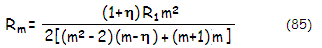The relation shows that, for given primary ƒ-ratio and secondary magnification, best (median) image surface is somewhat less curved in the Gregorian (see graph). This is despite its considerably stronger Petzval surface curvature, due to its astigmatism, opposite in sign to its Petzval, resulting in the astigmatic surfaces forming on the convex side of the Petzval, thus less curved relative to it. In the Cassegrain, astigmatic surfaces form on the concave side of its Petzval surface, with their curvatures consequently stronger (note that Petzval surface is concave toward secondary in the Cassegrain, and convex in the Gregorian).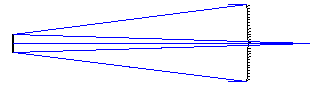EXAMPLE: 300mm ƒ/4/16 Classical Cassegrain, thus with numerically positive secondary magnification m=4; opting for the back focal distance in units of the primary's focal length η=0.2 (240mm), the height of marginal ray at the secondary, in units of the aperture radius, k=(1+η)/(m+1)=0.24. With the paraboloidal primary (K1=-1), the secondary conic for corrected spherical aberration is
K
2=-[(m+1)/(m-1)]2=-2.777.

Alternatively, secondary conic can be found from K2=-[(R2/i2)-1]2 which, for the secondary mirror radius
R
2=-mkR1/(m-1)=-768mm, and the secondary-mirror-to-primary's-image separation i2=kƒ1=-288, also gives K2=-2.777.

The wavefront error of coma is identical to that of a 300mm ƒ/16 paraboloid; at 0.25° (21mm, 0.00436 radians) off-axis, it is Wc=αD/48F2=0.0001065, or 0.194 wave P-V in units of 550nm wavelength. The RMS wavefront error of coma is smaller by a factor of 1/32, or 0.034 wave. At the same distance off-axis, astigmatism is Wa=-(m2+h)Dα2/8(1+h)mF=0.00015mm, or 0.27 wave P-V in units of 550nm wavelength; the corresponding RMS wavefront error is 0.056.

With R1=-2400mm and R2=ρR1=mkR1/(m-1)=0.32R1=-768mm, the Petzval curvature is Rp=-565mm, and the best (median) field curvature is Rm=-315mm.

# 8.2.3. Aplanatic two-mirror telescopes

It wasn't until 1910, when Ritchey in the U.S. and Chrétien in France arrived at needed conics for a coma-free two-mirror (Cassegrain) system, that the "classical" two-mirror telescope finally was upgraded to its optimal version. It took another 17 years before the very first such telescope was successfully made (Ritchey, 1927).

Correction of coma in a two-mirror system requires an additional, relatively small modification of both optical surfaces. In the Cassegrain configuration, secondary needs to be more strongly aspherised in order to correct for the coma. So does the primary, in order to compensate for additional spherical under-correction induced by the more strongly aspherised, aplanatic secondary. In the Gregorian, however, both mirrors need to be less strongly aspherised than in the classical arrangement. Needed conics for an aplanatic two-mirror telescope are: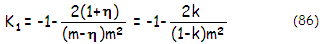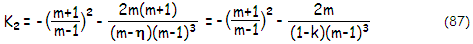Alternatively, the conics can be expressed in terms of the primary focal length ƒ1, system focal length ƒ, and mirror separation s as K1=-1-2ƒ12(ƒ1-s)/sƒ2 and K2=-[(ƒ-ƒ1)/(ƒ+ƒ1)]2-2ƒ[ ƒ1/(ƒ+ƒ1)]3/s. Note that ƒ1 is always numerically negative, and ƒ is numerically negative in the Gregorian, a result of Eq. 76.

With Seidel spherical aberration and coma corrected, the remaining aberrations are astigmatism, field curvature and distortion. The P-V wavefront error of Seidel astigmatism is given by: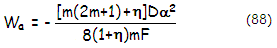This gives astigmatism in the aplanatic Cassegrain - also known as Ritchey-Chrétien - greater, and in the aplanatic Gregorian smaller by a factor of (2m2+m+η)/(2m2+η) compared to the classical types. Neglecting distortion, the remaining aberration is best, or median field curvature, given as: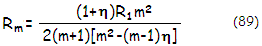Again, for an equal set of parameters, aplanatic Cassegrain has somewhat stronger best field curvature than aplanatic Gregorian. Graphs below illustrate the P-V error of astigmatism (left) and best image curvature (right) in the aplanatic two-mirror system. Note that the system focal length for the Gregorian is numerically negative, thus its median surface has positive sign, i.e. it is convex toward secondary; median surface in the Cassegrain has negative curvature, i.e. it is concave toward secondary. When expressed in units of primary's radius of curvature (R1, dashed), which has a constant negative sign, plots for the two configurations are of opposite sign (since the system focal length ƒ=mR1/2, the ratio of image curvature in units of R1 vs. that in units of the system f.l. is m/2).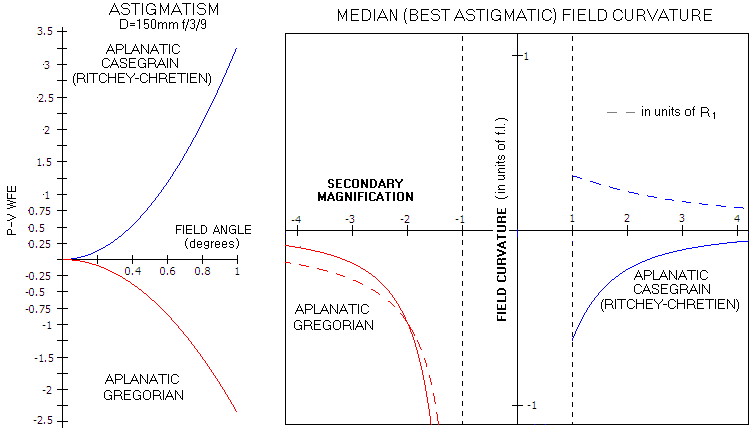Aplanatic Cassegrain has somewhat stronger, and aplanatic Gregorian somewhat weaker than classical arrangements, but the difference is small. Median field curvature of the aplanatic systems are nearly identical to those of the classical Cassegrain and Gregorian for the practical range of magnifications.

Till recent, most of large professional telescopes were of Ritchey-Chrétien type. Probably the most famous, Hubble Space Telescope, has 2.4m ƒ/2.3 primary mirror, at 4.9m from the convex secondary, and ƒ/24 effective system focal ratio. This gives all the information needed to specify its inherent aberrations: secondary magnification m=24/2.3=10.435, relative minimum secondary size k=(5.52-4.9)/5.52=0.112, and back focal distance in units of the primary focal length η=(m+1)k-1=0.28. This gives the primary mirror conic K1=-1.0023, secondary conic K2=-1.497, P-V wavefront error of astigmatism at the edge of its 9 arc minutes (0.15°) "data field" radius of 1.3 waves (λ=550nm), and the best image surface curvature radius Rm=-633mm.EXAMPLE: 300mm ƒ/3/12 aplanatic Gregorian, thus with numerically negative secondary magnification m=-4; opting for the back focal distance in units of the primary's focal length η=0.25 (225mm), the height of marginal ray at the secondary, in units of the aperture radius, k=(1+η)/(m+1)=-0.417, and the ratio of curvature radii for the two mirrors R2/R1=ρ=-0.3336. The aplanatic conic for the primary, compensating for spherical aberration induced by the aplanatic secondary K1=-0.963, and the secondary conic for corrected coma is K2=-0.405.

The only remaining point-image aberration is astigmatism, with the P-V wavefront error at 0.25° off-axis of -0.000165mm, giving -0.3 wave in units of 550nm wavelength, with the corresponding RMS wavefront error of 0.061 wave (the P-V error is of opposite sign to that in a Cassegrain).

With R1=-1800mm and R2=ρR1=mkR1/(m-1)=-0.334R1=600mm, the Petzval curvature is Rp=225mm, and the best (median) field curvature is Rm=349mm.